# Geospatial Network Visualization

{sfnetworks} meets {ggraph}

2021-03-05

# Welcome to my website!

This is the first entry for my brand new website built with `distill`. I hope you find this and hopefully the coming posts useful! So let’s get to it!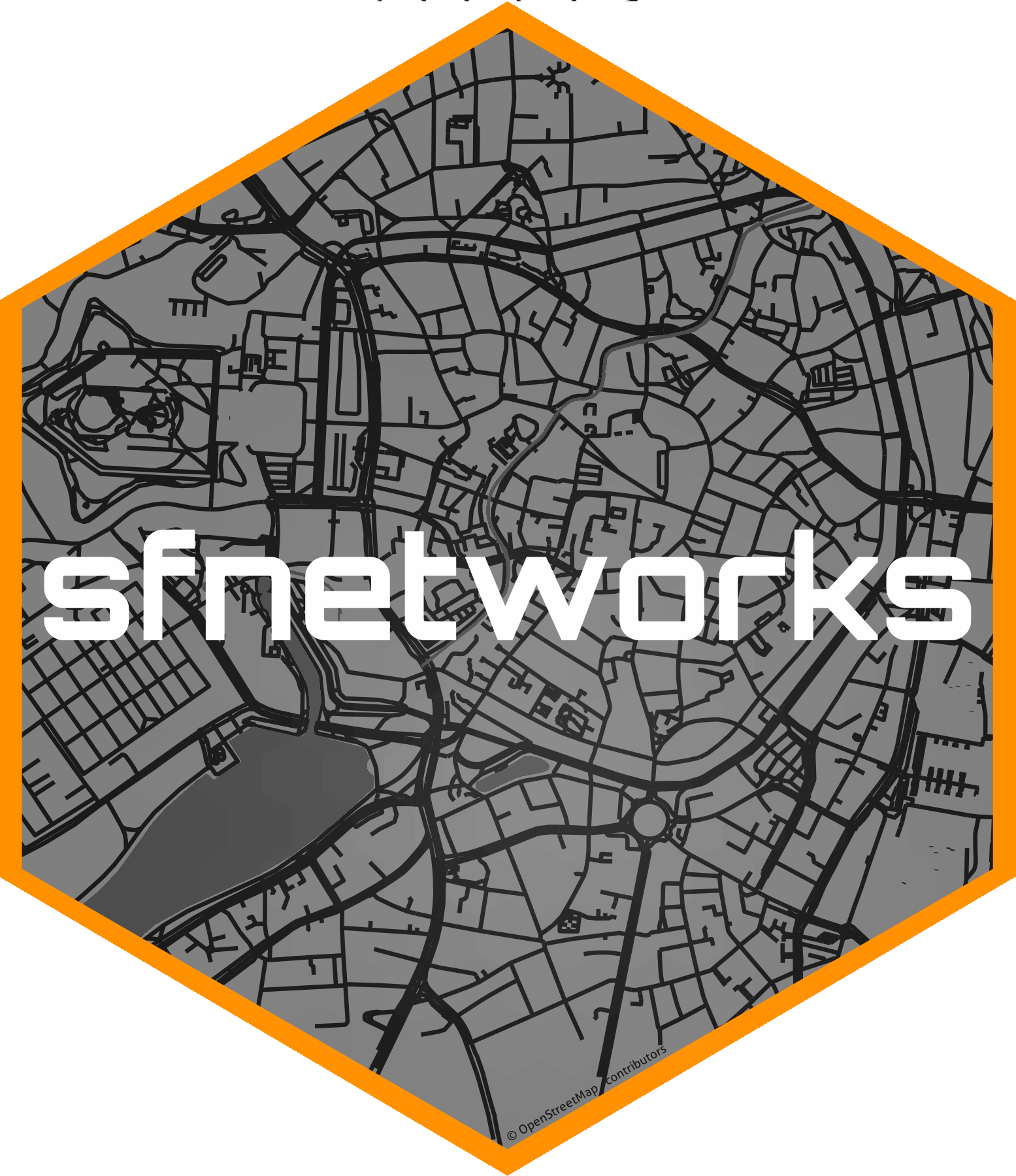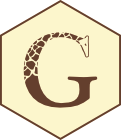This post is to document my personal exploration on how to visualize spatial networks created with `sfnetworks` using `ggraph`. This all started with a sfnetworks hackathon and should end with a successful pull request (PR) to `ggraph`.

I should start by saying, that it is probably a good idea to get familiar with `ggraph` (and `tidygraph` and `sfnetworks` while you are at it!) before going through this post.

If you are already a `ggraph` + `tidygraph` user and would like to apply the possibilities to the spatial domain, then this is definitely a resource for you and a good opportunity to learn about sfnetworks!

# What can be done?

There are already several possibilities to use sfnetworks and ggraph together. Why? you may be wondering. Well because ggraph was built as a way to visualize `tbl_graph`s from tidygraph, and guess what? `sfnetwork` objects sub-classes `tbl_graph`. Let’s look at the `roxel` demo dataset from sfnetworks.

``````library(sfnetworks)
library(sf)
net = as_sfnetwork(roxel, directed = F)
class(net)
``````
`` "sfnetwork" "tbl_graph" "igraph"   ``

So basically you can do all sort of crazy graph representations with an `sfnetwork` already.

The real aim of this integration is to allow you to do all this crazy graph representations + graph representations in geographical space! Not everything is possible yet, and you will see below some limitations.

For now I will illustrate how, with the current status quo, we can already integrate `sfnetworks` and `ggraph` for spatial network visualizations. So let’s get started!

# The Three Pillars

We need to start with three main concepts, the essential elements to create any `ggraph` visualization:1. Layouts contain the vertical and horizontal placement of the nodes, giving them a physical placement. In spatial terms, they contain the coordinates of where each node should be mapped.

2. Nodes refer to which representation the nodes should have. And this really refers to which visual representation they should have, known in `ggplot2` as `geom`. Should they be points, tiles, voronoi polygons? `ggraph` contains a large list of node representations via `geom_node_*()`.

3. Edges refer to the way nodes are connected between each other visually. Again here we are talking about `ggplot2` geometries and the `geom_edge_*()` functions should give you a big pool of options to represent this.

Let’s go through the possibilities to combine these elements with `sfnetwork` objects.

## 1. `layout_sf()`

As mentioned before, a layout is basically the physical representation of where to place our nodes. When we are dealing with non-spatial network representations we must remember that the nodes are not meant to represent actual locations on Earth, but can be placed anywhere. ggraph provides several algorithms to make this happen. Let’s take one of the examples in the ggraph layout vignette to illustrate. This will include the layout + nodes + edges in a non-geographical representation.

``````library(ggraph)
library(tidygraph)
library(tidyverse)
ggraph(
filter(net, group_components() == 1),
layout = 'focus',
focus = node_is_center()
) +
ggforce::geom_circle(aes(x0 = 0, y0 = 0, r = r), data.frame(r = 1:5), colour = 'grey') +
geom_node_point() +
coord_fixed()
``````But when talking about geospatial networks, we would usually want to place the nodes in their geographical location. A really nice thing about `ggraph`, is that you can give your own customized layout, by passing custom X and Y coordinates for node placement!

In sfnetworks, we have a spatial network consisting of nodes and edges. Since we have a nice integration with `sf`, we can extract the coordinates of our nodes as an X and Y coordinate dataframe, which we can pass to `ggraph`. Let me give you a quick demo instead of all these words.

``````net
``````
``````# A sfnetwork with 701 nodes and 851 edges
#
# CRS:  EPSG:4326
#
# An undirected multigraph with 14 components with spatially explicit edges
#
# Node Data:     701 x 1 (active)
# Geometry type: POINT
# Dimension:     XY
# Bounding box:  xmin: 7.522622 ymin: 51.94151 xmax: 7.546705 ymax:
#   51.9612
geometry
<POINT [°]>
1 (7.533722 51.95556)
2 (7.533461 51.95576)
3 (7.532442 51.95422)
4  (7.53209 51.95328)
5 (7.532709 51.95209)
6 (7.532869 51.95257)
# ... with 695 more rows
#
# Edge Data:     851 x 5
# Geometry type: LINESTRING
# Dimension:     XY
# Bounding box:  xmin: 7.522594 ymin: 51.94151 xmax: 7.546705 ymax:
#   51.9612
from    to name        type                                geometry
<int> <int> <chr>       <fct>                       <LINESTRING [°]>
1     1     2 Havixbecke~ reside~ (7.533722 51.95556, 7.533461 51.955~
2     3     4 Pienersall~ second~ (7.532442 51.95422, 7.53236 51.9537~
3     5     6 Schulte-Be~ reside~ (7.532709 51.95209, 7.532823 51.952~
# ... with 848 more rows
``````
``````net %>% st_coordinates() %>% head()
``````
``````         X        Y
1 7.533722 51.95556
2 7.533461 51.95576
3 7.532442 51.95422
4 7.532090 51.95328
5 7.532709 51.95209
6 7.532869 51.95257``````

Now, how do we create a layout that extracts the coordinates automatically? Well here is a little helper function that will take care of that for you. It will extract the X and Y coordinates of any `sfnetwork` object into a data.frame that is understood by `ggraph()`.

``````layout_sf = function(graph){
# Extract X and Y coordinates from the nodes
graph = activate(graph, "nodes")
x = sf::st_coordinates(graph)[,"X"]
y = sf::st_coordinates(graph)[,"Y"]
data.frame(x, y)
}
``````

How do we use it? Well, like this:

``````library(ggraph)
ggraph(net, layout = layout_sf)
``````Yes! That’s it. You are probably looking now at a beautiful blank canvas, but internally `ggraph()` has already a plan for every node you will map next, let’s see it in action in the next step.

## 2. `geom_node_*()`

The most obvious way to represent a node in space is with a point. This is how we would do it if we were plotting `POINT` geometries in space. With ggraph we can get that representation with `geom_node_point()`. Note that other useful node representations in space might be using a label or text. We can achieve that with `geom_node_label()` and `geom_node_text()`, respectively.

Building on our previous plot:

``````ggraph(net, layout = layout_sf) +
geom_node_point()
``````Oh yes, there are our nodes! But wait… If you are familiar with the sfnetworks vignettes, you might be thinking: this looks distorted… and yes, it does.

Unfortunately, `ggraph()` does not know about coordinate reference systems (CRS) so it will accommodate your X and Y axes to the size of your plot. This is one of the reasons why some internal tweaks are needed in ggraph to make this work better. But for now a way to go around this is to use `coord_sf()`:

``````ggraph(net, layout = layout_sf) +
geom_node_point() +
coord_sf(crs = st_crs(net))
``````Much better. Now, our plot also takes into consideration the CRS and places our nodes properly.

Now let’s give some aesthetics to our plot. If you scroll back up, you will see that our nodes don’t have attributes, other than their geometry, so what should we look at? What about the degree centrality of the node? This will calculate the number of incident edges for each of our nodes. We can do this with the `centrality_degree()` function in `tidygraph`.

A really nice feature about `ggraph` is that we don’t need to go back to our original graph, mutate our network, save as a new object, and then call it again inside `ggraph()`. We can just call the function directly inside the `aes()`, where the calculation will be done on the fly! Read more about it here.

``````library(tidygraph)
ggraph(net, layout = layout_sf) +
geom_node_point(aes(color = centrality_degree())) +
coord_sf(crs = st_crs(net))
``````Directly passing functions also works inside `facet_*()` functions. sfnetworks has a couple of functions that can be evaluated in this way. To illustrate we can use `node_X()`, which gives us the X coordinate of the nodes.

``````ggraph(net, layout = layout_sf) +
geom_node_point(aes(color = centrality_degree())) +
coord_sf(crs = st_crs(net)) +
facet_nodes(~node_X() > 7.535)
``````OK, probably not a real-world case scenario, but it gives an overview of what can be done!

Let’s move on to the final step, and connect these nodes to each other.

## 3. `geom_edge_*()`

Now comes the tricky part. When we are working with graph structures in, let’s call it, “abstract” space, the connections between the nodes are basically straight lines between each `from` and `to` pair. Although `ggraph` has quite a long list to represent edges (see here), the connections that I find most relevant for spatial networks are `geom_edge_link()` and `geom_edge_arc()`, which create a straight line or an arc between connected nodes.

``````ggraph(net, layout_sf) +
geom_edge_arc() +
geom_node_point() +
coord_sf(crs = st_crs(net))
``````Yes, I bet you are thinking this could go to accidental aRt. But depending on the visualization you are creating, this `geom` can come in handy for some data cases, you can see it in action at the end of this post!

But now let’s look at straight lines or links between our nodes. We can of course pass aesthetics to all the `geom_edge_*()` functions, which refer to edge attributes. Let’s color our edges by the type of road:

``````ggraph(net, layout_sf) +
geom_node_point(size = 0.75) +
coord_sf(crs = st_crs(net))
``````I know that for now you must be wondering: if we have a spatial set of edges with an explicit geometry, why are we just drawing plain lines? Well, remember I said this would be tricky? This is exactly what is missing from ggraph and the core of what I eventually want to implement.

But, do not despair! I am here to show you some workarounds, not fully ideal but something to work with in the meantime. Let’s remember that ggraph subclasses a `ggplot` object, so we can combine ggplot2 functions, and any other package that extends the grammar of graphics.

We will resort to `geom_sf()` for now. We can plot the edges of our network, by extracting them as an `sf` object with the function `st_as_sf()`. We have implemented a shortcut that allows you to choose which element of the network (nodes or edges), you want to activate and extract.

``````ggraph(net, layout = layout_sf) +
geom_sf(data = st_as_sf(net, "edges")) +
geom_node_point() +
coord_sf(crs = st_crs(net))
``````And there we go, a nice representation of a geospatial network! We can of course pass some aesthetics as well, for example an spatial edge predicate implemented in sfnetworks: `edge_circuity()`. You will see now that we can pass these predicates directly to the aesthetics inside `geom_sf()` and since our main object is a `ggraph` this expression will be evaluated in the network, pretty exciting!!

``````ggraph(net, layout = layout_sf) +
geom_sf(
data = st_as_sf(net, "edges"), size = 0.8,
aes(color = as.numeric(edge_circuity()))
) +
scale_color_viridis("Circuity") +
coord_sf(crs = st_crs(net))
``````And there you have it, a swift overview of how to use `ggraph` and `sfnetworks` together.

# What can’t be done?

So yes, a lot can be done already, but as you may have noticed, there are certain things that just don’t work yet with the current `ggraph` implementation. Here is a not at all comprehensive list of things that need some work:

• The `layout_sf()` function I showed you above will not work when there are columns named `x` or `y`.
• `ggraph()` does not consider the network CRS.
• There is no way yet to plot spatially explicit edges inside ggraph.

This last one comes with a couple more problems:

### Multiple scales for the same aesthetic

With `ggraph` one can give “color”, “fill”, “size/width”, etc. aesthetics to both the nodes and the edges. In our current workaround this is not working so good. The plot will get rendered properly with the corresponding colors, but the legend does not know what to do, and will only use one of the scale elements, without a warning!

``````ggraph(net, layout = layout_sf) +
geom_sf(
data = st_as_sf(net, "edges"),
aes(color = as.numeric(edge_circuity()))
) +
geom_node_point(
aes(color = centrality_betweenness())
) +
coord_sf(crs = st_crs(net))
``````### Faceting edges

Another missing stone is faceting by edges. This currently gives an error:

``````ggraph(net, layout = layout_sf) +
geom_sf(data = st_as_sf(net, "edges")) +
facet_edges(~type)
``````
``Error in seq_len(length(data) - 1): argument must be coercible to non-negative integer``

What to do then? Remember that I mention a PR? Well, I am working there to fix these issues, some of them already have a fix, others don’t. I would certainly appreciate any help I can get. I opened an issue to illustrate the progress of my PR. Basically I am stuck with understanding `ggproto` objects to allow an integration of edges in geographical space.

If you feel like exploring what I have got so far, install `ggraph` from my forked repo. Beware, this will replace the original `ggraph`, so do this under your own risk.

``````remotes::install_github("loreabad6/ggraph")
``````

# Show me more!

While testing my `ggraph` implementation, I started looking for some spatial network visualization examples done with R that I could recreate with my code. Here I added two examples of what I came up with, and will probably add more to this GitHub repository if you are interested in following it up. Bear in mind that most of these examples are created with `remotes::install_github("loreabad6/ggraph")`.

### Break Free from Plastic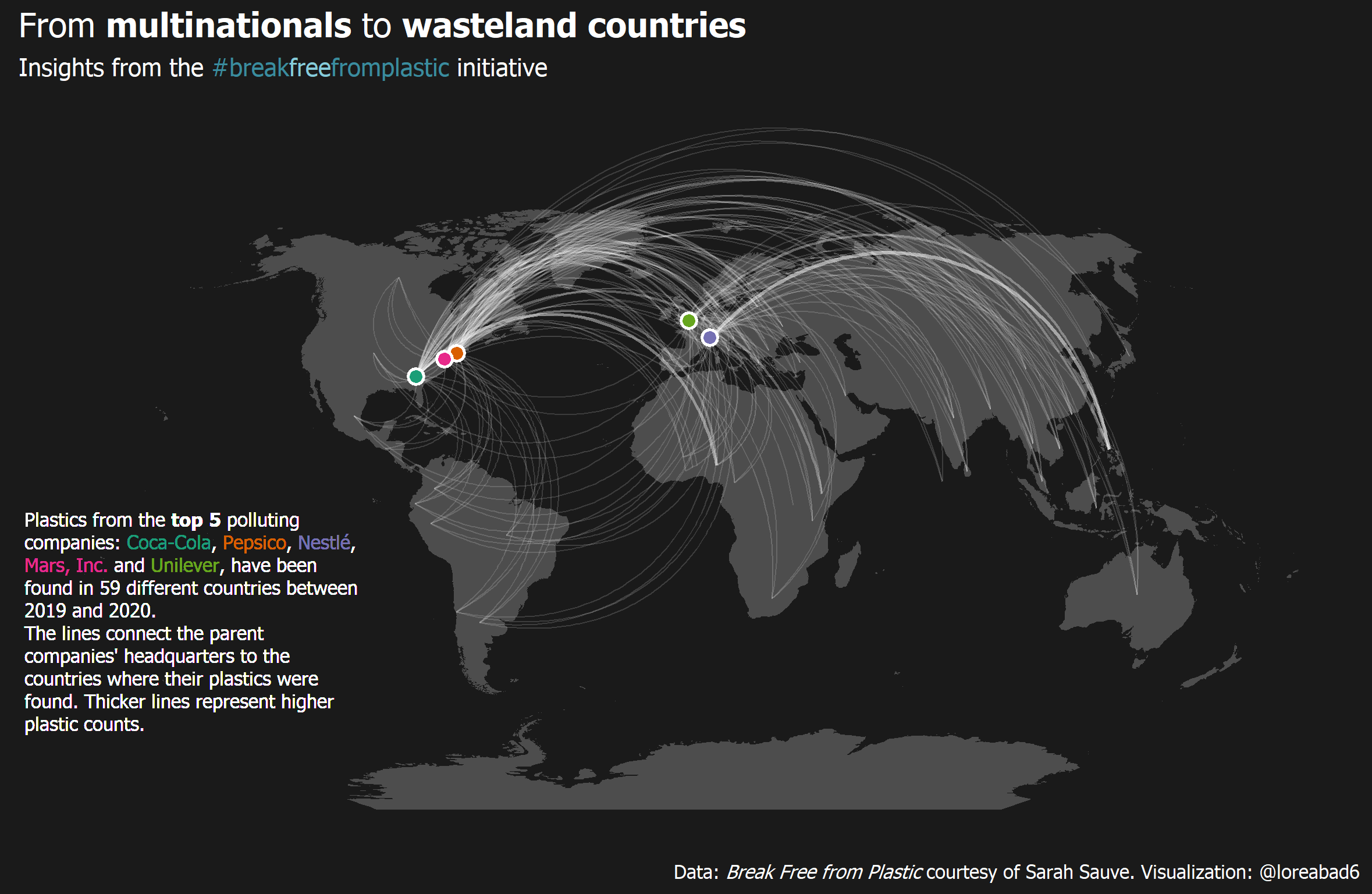### Airports in the U.S.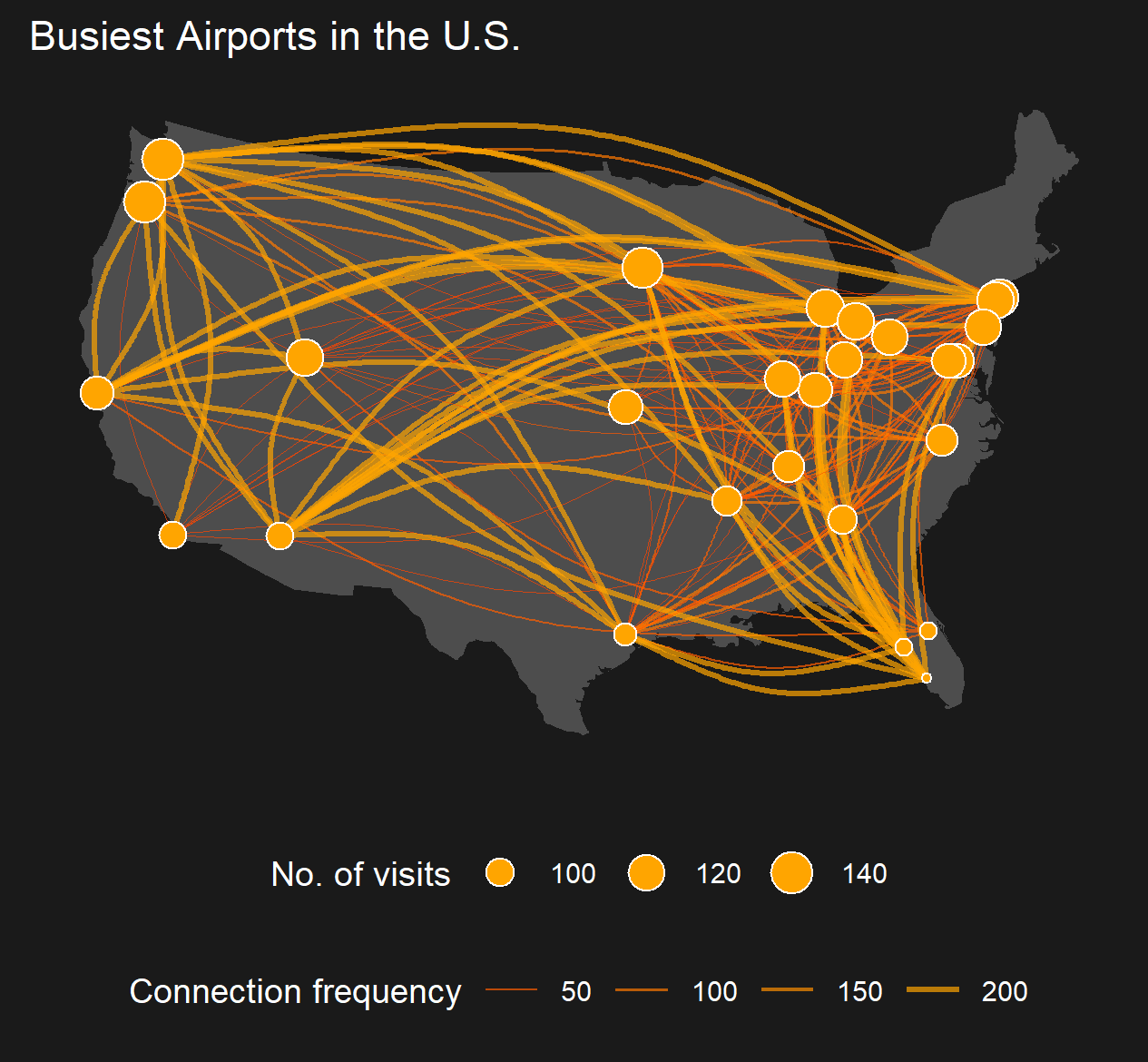### The fourth way of visualizing a graph on a map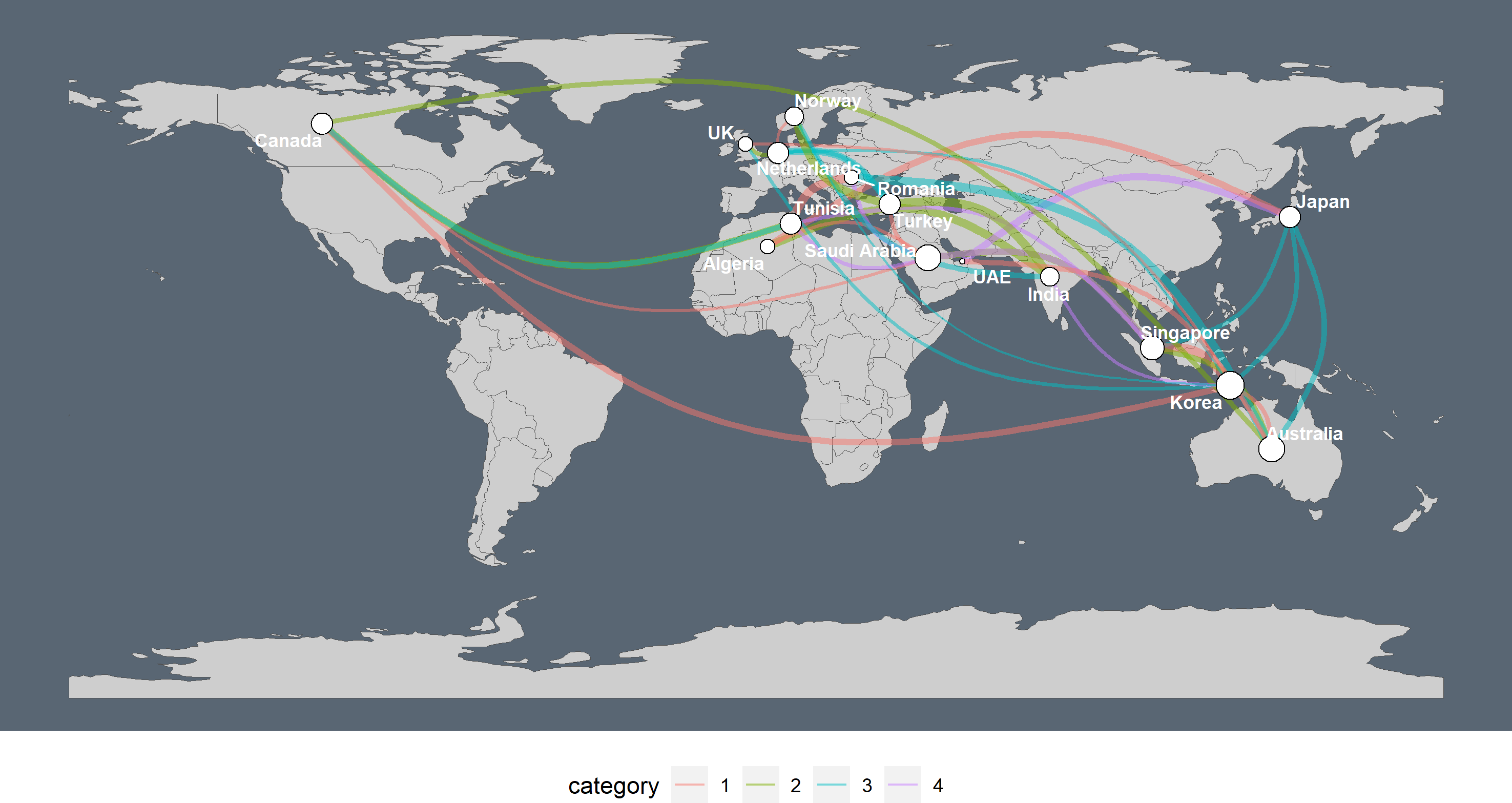### Corrections

If you see mistakes or want to suggest changes, please create an issue on the source repository.

### Citation

`Abad (2021, March 5). Lore Abad: Geospatial Network Visualization. Retrieved from https://loreabad6.github.io/posts/2021-03-05-geospatial-network-visualization/`
```@misc{abad2021geospatial,